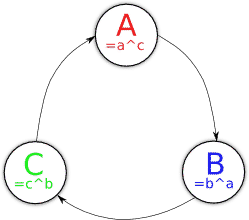# The Dining Cryptographer's Problem

From talking to students in Computer & Network Security at the University of Sydney, the dining cryptographer's problem can still cause question marks. It's understandable -- the protocol can look pretty confusing at first. Hopefully this helps short circuit the confusion =]

## Problem Definition

David Chaum introduced the problem in his paper The Dining Cryptographers Problem: Unconditional Sender and Recipient Untraceability:

Three cryptographers are sitting down to dinner at their favorite three-star restaurant. Their waiter informs them that arrangements have been made with the maitre d'hotel for the bill to be paid anonymously. One of the cryptographers might be paying for the dinner, or it might have been the NSA (U.S. National Security Agency). The three cryptographers respect each other's right to make an anonymous payment, but they wonder if the NSA is paying.

Is there some way to check the NSA haven't paid whilst keeping the benevolent cryptographer anonymous?

## The Protocol

Each cryptographer flips a coin (0 or 1), with the value of Alice's coin being a, Bob's being b and so on. Each cryptographer then checks if their coin's value is the same as the person's next to them (xor) and stores these computed values (A for Alice, B for Bob, ...).Finally, we xor all these computed values together. If everyone is telling the truth about their computed values, then the result should be zero as all the values cancel out.

A ^ B ^ C
= (a ^ c) ^ (b ^ a) ^ (c ^ b)
= a ^ c ^ b ^ a ^ c ^ b
= c ^ b ^ c ^ b
= c ^ c
= 0
[each pair of equivalent variables cancel out as it's xor]

If one of the cryptographers wishes to anonymously admit to paying for the meal however, they can flip the value of their computed result. If this was done, the result would be 1 but no-one would know who "lied" about their computed result. For example, if B had actually paid for the meal...

A ^ B ^ C
= (a ^ c) ^ ¬(b ^ a) ^ (c ^ b)
= (b ^ a) ^ ¬(b ^ a)
= X ^ ¬X
= 1

Thus, as long as the benevolent cryptographer flips their computed value:
• If the result is 0 then the NSA have paid for the meal
• If the result is 1 then a benevolent cryptographer has shouted his friends anonymously

Note however that this only works if a single cryptographer has paid for the meal and all the cryptographers act truthfully. If two cryptographers split the cost of the meal between them and both flipped their computed values then the values would cancel out and it would appear the NSA paid for the meal. These concerns are addressed by the anonymous veto network -- but that's a story for another day =]